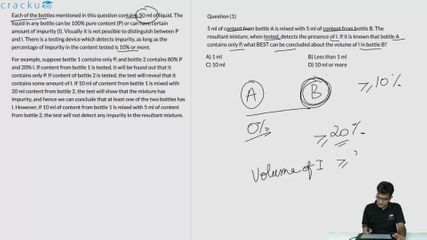### CAT 2021 Question Paper (Slot 3) Question 27

Instructions

Each of the bottles mentioned in this question contains 50 ml of liquid. The liquid in any bottle can be 100% pure content (P) or can have certain amount of impurity (I). Visually it is not possible to distinguish between P and I. There is a testing device which detects impurity, as long as the percentage of impurity in the content tested is 10% or more.

For example, suppose bottle 1 contains only P, and bottle 2 contains 80% P and 20% I. If content from bottle 1 is tested, it will be found out that it contains only P. If content of bottle 2 is tested, the test will reveal that it contains some amount of I. If 10 ml of content from bottle 1 is mixed with 20 ml content from bottle 2, the test will show that the mixture has impurity, and hence we can conclude that at least one of the two bottles has I. However, if 10 ml of content from bottle 1 is mixed with 5 ml of content from bottle 2. the test will not detect any impurity in the resultant mixture.

Question 27

# There are four bottles. It is known that three of these bottles contain only P, while the remaining one contains 80% P and 20% I. What is the minimum number of tests required to definitely identify the bottle containing some amount of I?

Solution

The percentage concentration of the impure solution is 80 percent.

When equal volumes of all four solutions are mixed.

Considering 10 ml of each we have impurity to be 2ml/40ml. The impurity concentration is less than 10 percent and hence cannot be recognized.

Similarly when equal volumes of one impure and 2 pure solutions are mixed.

The impurity in the solution is 2ml/30ml which is less than 10 percent and hence cannot be recognized.

Hence for detecting the impure solution we must use equal volumes of 2 solutions at a time.

Considering the three pure solutions to be P and the impure solution to be I.

P, P, P, I.

Considering equal volumes of solution from the bottle one bottle of P, and I. Testing this would recognize the impurity.

After this consider one bottle among the other 2 P bottles which are left and test this with one among the previously tested P, I.

If the one considered is I it will detect the impurity and confirms the bottle to be I.

If the one considered is P it will fail to detect the impurity and hence the other bottle will be I.

Hence a minimum of two tests are required to identify the bottle with the impurity.

### View Video Solution